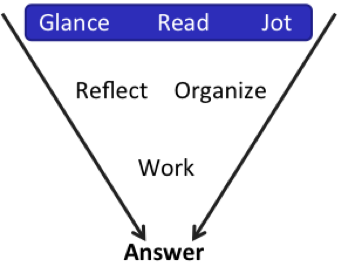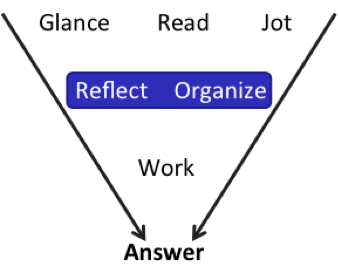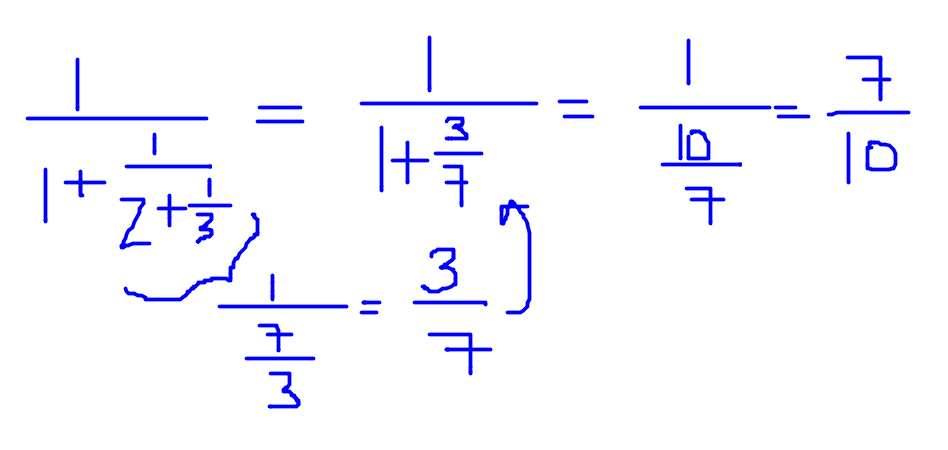# More Fast Math for the GMAT - Part 6

by , Aug 6, 2017A while back, we started a series on Fast Math for the GMATand weve got more for you today!

On these two new problems, were going to employ some broader principles than the ones we saw in the earlier installments of this series. I wont say any more yettry the two problems from the free problem set that comes with GMATPrep and then well talk.

Set your timer for 4 minutes and go!

[pmath]1/{1+{1/{2+1/3}}}[/pmath]

(A) [pmath]3/10[/pmath]

(B) [pmath]7/10[/pmath]

(C) [pmath]6/7[/pmath]

(D) [pmath]10/7[/pmath]

(E) [pmath]10/3[/pmath]

The Earth travels around the Sun at a speed of approximately 18.5 miles per second. This approximate speed is how many miles per hour?

(A) 1,080

(B) 1,160

(C) 64,800

(D) 66,600

(E) 3,996,000

Well talk about the first one in this installment and the second one in the next installment.Glance: Wow, that fraction is ugly! Glance down at the answers, too. Notice anything?

Answers (A) through (C) are less than 1 and answers (D) and (E) are greater than 1. Is there a way to tell whether its greater or less than 1? Also, Answers (A) and (E) are mirror images and so are (B) and (D). That makes sense, because chances are the most common trap answer will be someone solving correctly but just reversing the fraction by accident. Answer (C) doesnt have a mirrorso if I have to guess, Im not going to guess that. (And, in fact, if I solve and get (C), I might actually check my work.)Hmm. Im going to go back and reflect on my first thought about greater / less than 1. The top of the main fraction is the number 1. The bottom of the main fraction is 1 + something. That something is positive, so the overall fraction is 1 over something a little bigger than 1.

Is that going to be greater than 1 or less than 1?

1 over (>1) is less than 1. Eliminate answers (D) and (E).

From here, you can just straight up solve. If youre confident that (C) isnt going to be right, though, you can also estimate. Why? Because answer (A) is[pmath]3/10[/pmath] and answer (B) is [pmath]7/10[/pmath]. Those are pretty far apartlike 30% and 70%.

[pmath]1/{1+{1/{2+1/3}}}[/pmath]

Look at that thing again. 2 + [pmath]1/3[/pmath] is about 2 (or close enough!). So the bottom part of the fraction is about 1 +[pmath]1/2[/pmath] = [pmath]3/2[/pmath].

And then 1 over[pmath]3/2[/pmath] just means take the reciprocal, which is [pmath]2/3[/pmath].

You might be thinking, sure, I see how that works, but the actual math isnt all that hard so why not just do it?

Heres why: When Im studying Im not just looking for ways to get this problem right. Im also looking for ways to approach harder problems of the same type. I might get one that looks like this but has way harder math and, on that other one, I might not notice that I can just estimate because Im so intimidated by the scary / annoying math.

In short: I can learn a lot on easier problems by brainstorming alternative approaches and not just resting on textbook math because the maths not that hard on this problem.

By the way, here is the math.Im not saying that you shouldnt solve it this wayIm saying that there are other things to learn from this problem than just how to do this math.

## Key Fast Math Takeaways:

(1) You can estimate a lot more than you might think on the GMAT. If the question stem asks for an approximate answerof course, estimate. But, on PS, also glance at those answers before you begin to solve. Certain characteristics can indicate a good opportunity to estimate.

(2) What kinds of characteristics? The most common one is simply answers that are spread out. You can also add to that answers that fall on either side of some dividing linefor example, some are greater than 0 and some are less than 0. Some are more than 1/2 and some are less than 1/2 (that one is especially good for probability questions!). And so on.

* GMATPrep questions courtesy of the Graduate Management Admissions Council. Usage of this question does not imply endorsement by GMAC.